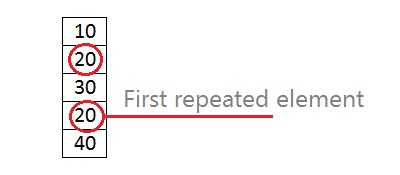# C program to find the first repeated element in an array

This program will read 5 integer numbers and find first repeated element, program will print the element and its index on which repeated element found.

This program will read an integer one dimensional array and find the first repeated element. Let suppose there are 5 elements in an array 10, 20, 30, 20, 40. Here element 20 repeated at 3rd index (count starts from 0).Logic:

We have to use two loops (nested loops), let check first element to other elements, if same element found, get the index and break the loop, run the loop until same element is not found or end of the elements.

## Program to find first repeated element in C

```#include <stdio.h>

int main()
{
int arr;
int i,j,n=5;
int ind,ele; //to store index & element

for(i=0; i<n; i++)
{
printf("Enter element %d: ",i+1);
scanf("%d",&arr[i]);
}

printf("Array elements are: ");
for(i=0; i<n; i++)
printf("%d ",arr[i]);

printf("\n");
ind=-1;
//check first repeated element
for(i=0; i<n; i++)
{
for(j=i+1; j<n; j++)
{
if(arr[i]==arr[j])
{
ele=arr[j];
ind=j;
break;
}
}

if(ind != -1)
break;
}
if(ind!=-1)
printf("%d repeated @ %d index\n",ele,ind);
else
printf("There is no repeated element\n");

return 0;
}
```

Output

```Firsr run:
Enter element 1: 10
Enter element 2: 20
Enter element 3: 30
Enter element 4: 20
Enter element 5: 40
Array elements are: 10 20 30 20 40
20 repeated @ 3 index

Second run:
Enter element 1: 10
Enter element 2: 20
Enter element 3: 30
Enter element 4: 40
Enter element 5: 50
Array elements are: 10 20 30 40 50
There is no repeated element
```